# 关于循环语句中for语句，switch语句，do-while语句的使用与区别

for语句，while语句，do-while语句这三种语句是C语言中的条件语句，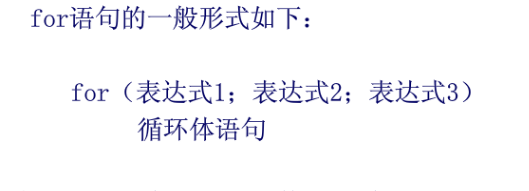for语句流程图：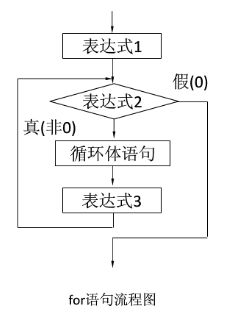#include <stdio.h>
int main()
{
int sum = 0;
for (int start = 1; //初始化
start <=100;    //条件控制
++start         //循环变量增加
)
{
sum += start;
}
printf("结果:%d\n", sum);
return 0;
}

for语句最大的特点将循环三要素都设置在控制结构，这是非常方便的。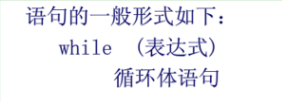while语句流程图：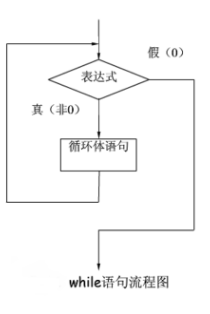注：while循环中的循环初始值，只能在while循环语句前编写。

while语句通常就是用在一些很难计算出循环次数的语句之中，比较灵活。相同的例子：

#include <stdio.h>
int main()
{
int sum = 0;
int start = 1;//初始化
while (start <=100)//条件判断
{
sum += start;
++start;//循环变量增加
}
printf("结果:%d\n", sum);
return 0;
}

do-while语句比较特别，是先执行循环体语句，再进行判断，即最少执行一次；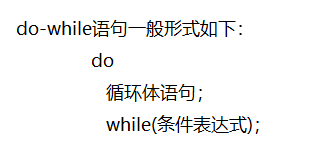do-while的结构图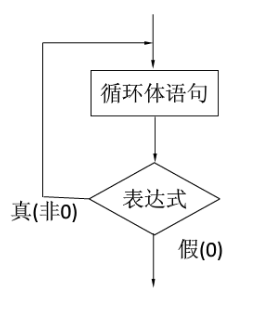#include<stdio.h>
int main()
{
int sum = 0;
int start = 1;
do
{
sum += start;
start++;
}while(start <= 100);
printf("结果：%d",sum);
return 0;
} 

if是适合用于判断条件是一个范围的情况，也就是知道循环次数；

while语句在表达方式上更自由灵活，适用于无法事先判断循环次数的循环

if和while都是要先进行判断，在进行循环。

do-while是先循环一次，在语句末尾进行判断，是否循环。不论最后是否循环，其中的语句最少会被执行一次。